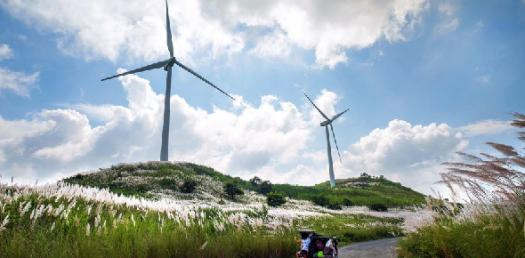# Wind Turbine Exam W/ Bernoulli Principal

25 Questions | Attempts: 238Settings.

• 1.
According to Bernoulli’s principle if velocity increases pressure _____________?
• A.

Increases

• B.

Decreases

• C.

Does Nothing

• D.

None of the Above

• E.

I Don't Know

• 2.
According to Bernoulli’s principle if pressure increases then velocity must ______.
• A.

Decrease

• B.

Increase

• C.

Does Nothing.

• D.

None of the Above

• E.

I Don't Know

• 3.
If the pressure under the wing of an airplane is greater than the pressure on top of the wing, the airplane should ______.
• A.

Fall

• B.

Land

• C.

Lift

• D.

A and B

• E.

I Don't Know

• 4.
Energy an object has due to it's motion is______________.
• A.

Potential Energy

• B.

Kinetic Energy

• C.

Gravitational Energy

• D.

I Don't Know

• 5.
Two balls are rolling at the same speed. One ball weighs more than the other. The heavier ball will have a __________ amount of kinetic energy.
• A.

Greater

• B.

Less

• C.

Equal

• D.

I Don't Know

• 6.
A book is placed on a desk. As it falls off of the desk it is an example of ________________.
• A.

Kinetic energy converting into potential energy

• B.

Potential energy converting into kinetic energy

• C.

I Don't Know

• 7.
What form of energy is the desired output of the wind turbine?
• A.

Electrical Energy

• B.

Mechanical Energy

• C.

Solar Energy

• D.

Kinetic Energy

• E.

I Don't Know

• 8.
Wind turbines _______________ differnt forms of energy.
• A.

Convert

• B.

Create

• C.

Produce

• D.

I Don't Know

• 9.
This is an example of a ____________ axis wind turbine?
• A.

Vertical

• B.

Horizontal

• C.

I Don't Know

• 10.
Which of the following could be positive impacts of wind energy?(Hint: you can select more than one)
• A.

It is GREEN energy

• B.

It creates jobs

• C.

It is loud

• D.

It reduces our dependency on petroleum (gas & oil)

• E.

It can disrupt bird migration

• F.

It can reduce energy costs

• G.

I Don't Know

• 11.
Which one is a renewable energy
• A.

Coal

• B.

Oil

• C.

WInd

• D.

Natural Gas

• E.

I Don't Know

• 12.
Can Energy be Created
• A.

Yes

• B.

No

• 13.
Which of the following is a form of energy
• A.

Solar

• B.

Nuclear

• C.

WInd

• D.

All of the above

• E.

I Don't Know

• 14.
The Tech Design Process is NOT a continous loop of steps to be completed to help solve problems.
• A.

True

• B.

False

• 15.
Constructing a prototype comes before developing possible solutions.
• A.

True

• B.

False

• 16.
The "Testing and Evaluating" step consist of?
• A.

Implenting the soloution

• B.

Verifing if the solution works

• C.

Examining the effectiveness of the solution

• D.

All of the above

• E.

I Don't Know

• 17.
___________ is the first step in the design process.
• A.

Gather Information

• B.

Redesign & Communicate

• C.

Understand the Problem

• D.

I Don't Know

• 18.
Why is the Redesign & Communicate step of the design process important?
• A.

It helps to gather information about the problem.

• B.

It allows designs to be continually improved.

• C.

It helps to test design solutions.

• D.

I Don't Know

• 19.
A group of parts working together to achieve a desired goal is a _____________.
• A.

Cycle

• B.

Machine

• C.

System

• D.

Technology

• E.

I Don't Know

• 20.
A system with feedback is a open loop system.
• A.

True

• B.

False

• 21.
The order of a system is _____________.
• A.

Output --> Input --> Process

• B.

Input --> Process --> Output

• C.

Input --> Output --> Process

• D.

I Don't Know

• 22.
All  systems have both positive and negative outputs.
• A.

True

• B.

False

• 23.
Resources are part of which step in the System's Model
• A.

Input

• B.

Process

• C.

Output

• D.

Feedback

• E.

I Don't Know

• 24.
The spoiler of a car is shaped so that pressure stays on the _____ of the car.
• A.

Bottom

• B.

Top

• C.

Side

• D.

Opposite side

• E.

I Don't Know

• 25.
Bernoulli's Principal applies to which of the following?There is more than 1 correct answer, please check all that can apply.
• A.

Sailing

• B.

Flight

• C.

Wind Turbines

• D.

Automotive Racing

• E.

I Don't Know

## Related TopicsBack to top
×

Wait!
Here's an interesting quiz for you.